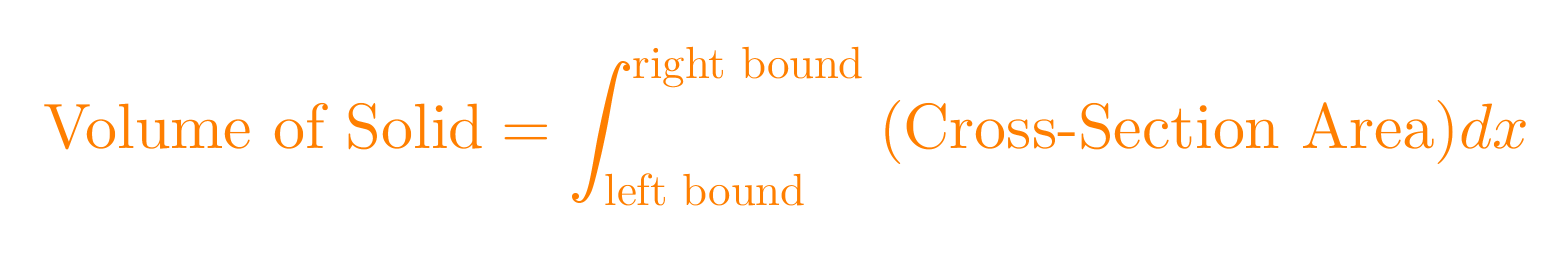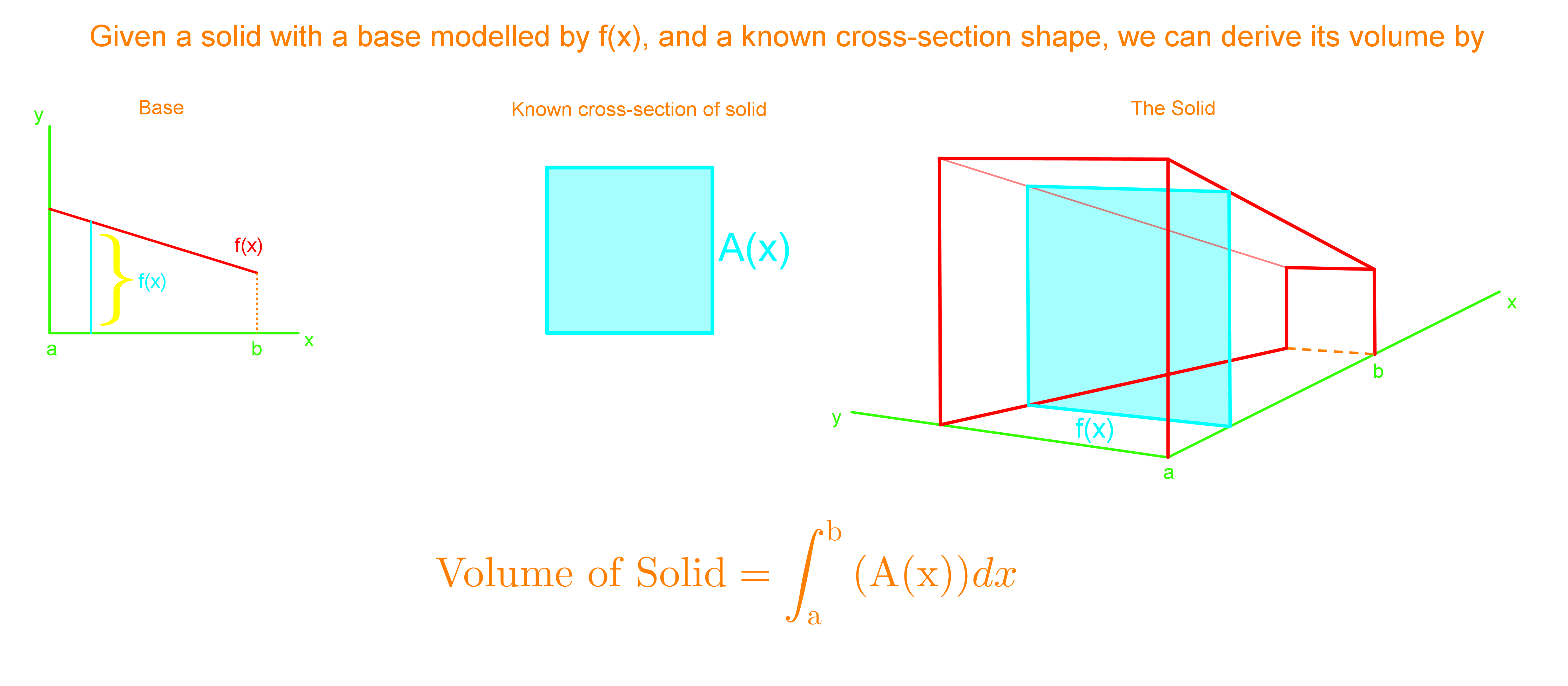# Volumes of solid with known cross-sections

### Volumes of solid with known cross-sections

In this section, we learn that cross sections are shapes we get from cutting straight through the curve. We want to find the area of that cross section, and then integrate it with known bounds to find the volume of the solid. In most cases they will tell you what the shape of the cross-section is, so that you can find the area of cross-sections immediately.

#### Lessons

Cross-Section Area of Solid = A(x)• Introduction

• 1.
A solid has a base bounded by $y=- {x\over2}+1$, $x=-2$,and $x=1$. If the parallel cross-sections perpendicular to the base are squares, find the volume of this solid.

• 2.
A solid has a base bounded by these two curves, y= sin x, y= cos x. If the parallel cross-sections perpendicular to the base are equilateral triangles, find the volume of this solid.